o
osc_pn11u1x9

# 查找子字符串

## Introduction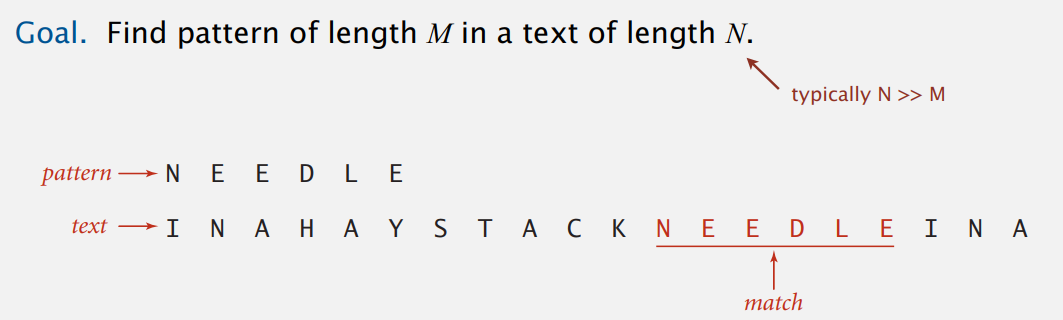## Brute Force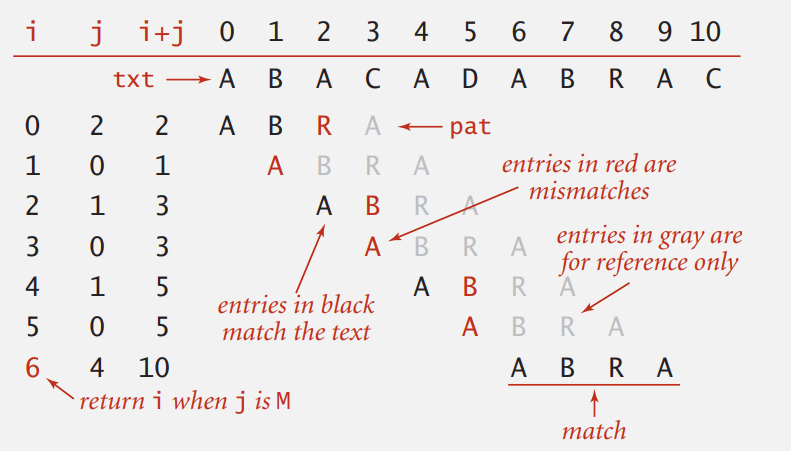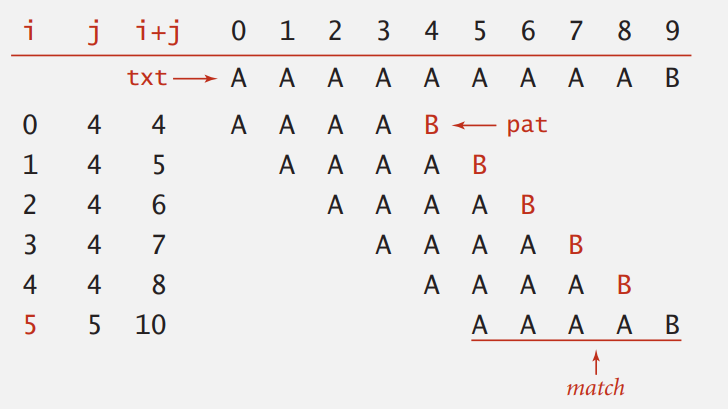``````public static int search(String pat, String txt) {
int M = pat.length();
int N = txt.length();
for (int i = 0; i <= N - M; i++) {
int j;
for (j = 0; j < M; j++)
if (txt.charAt(i + j) != pat.charAt(j))
break;
if (j == M) return i;    // index in txt where pattern starts
}
}
``````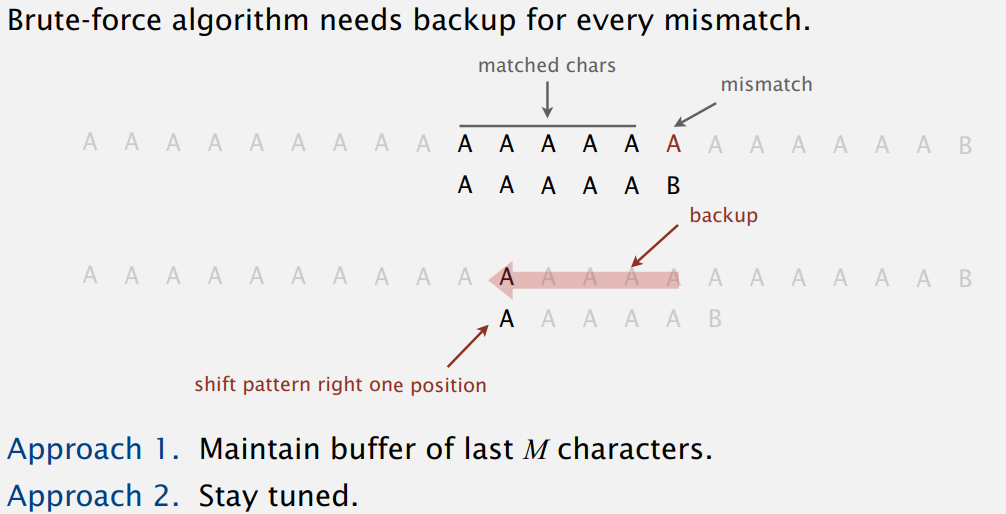``````public static int search(String pat, String txt) {
int i, N = txt.length();
int j, M = pat.length();
for (i = 0, j = 0; i < N && j < M; i++) {
if (txt.charAt(i) == pat.charAt(j)) j++;
else {
i -= j;    // explicit backup
j = 0;
}
if (j == M) return i - M;
}
}
``````

## Knuth-Morris-Pratt

KMP 算法一下子解决了上面两个问题，既不用回退，最多也只要访问 N 次字符，于是我们先来了解下 DFA

### Deterministic Finite State Automaton

• 状态数目是有穷的（包括初始状态和终结状态）。

• 每个状态对每个字符有且仅有一个转移。

• 转移到终结状态则接受这个字符串，即含有我们寻找的子串（模式）。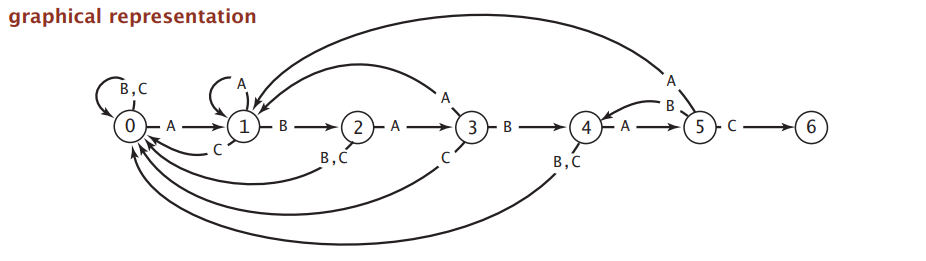``````           j    0    1    2    3    4    5
--------------------------
pat.charAt(j)   A    B    A    B    A    C    if in state j reading char c:
|A   1    1    3    1    5    1        if j is 6 halt and accept
dfa[][j]|B   0    2    0    4    0    4        else move to state dfa[c][j]
|C   0    0    0    0    0    6
``````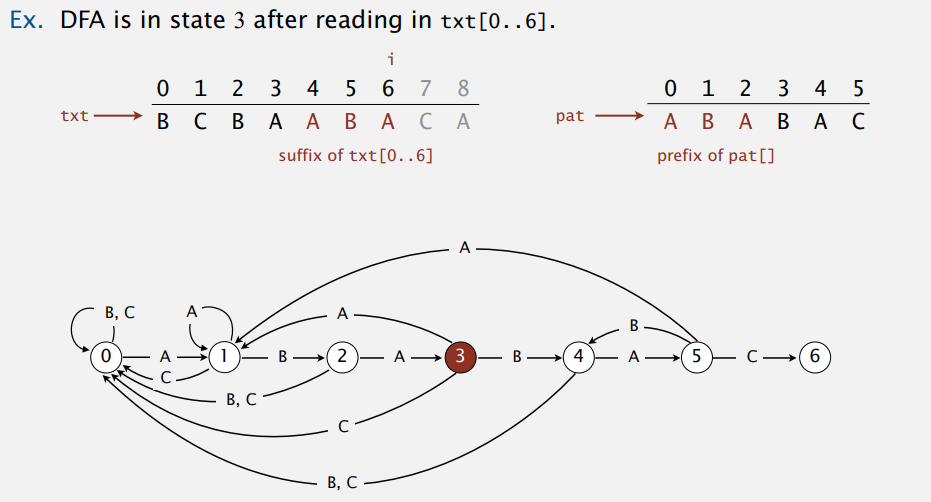### KMP Substring Search: Java Implementation

``````public int search(String txt) {
int i, j, N = txt.length();
for (i = 0, j = 0; i < N && j < M; i++)
j = dfa[txt.chaAt(i)][j];    // no backup
if (j == M) return i - M;
}
``````

### Construct DFA

1. Match Transition

匹配时的转移很好办，直接到下一个状态即可。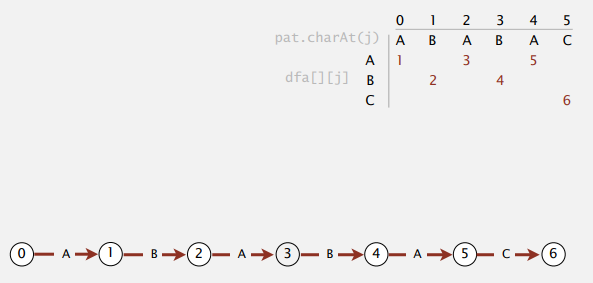2. Mismatch Transition

关键在不匹配时该如何转移。

假设在状态 j 时读到的下一个字符 c 不等于要找的子串的第 j + 1 个字符（pat.charAt(j)，从 0 标号），那么这个时候，我们从文本流中最近读出的 j - 1 个字符即为 pat[1..j - 1] + c，就是暴力算法要重新扫描的部分。

当前首字母到状态 j 出现了不匹配，按暴力算法该丢弃它从下一个字母再开始，即 pat，再一路重新扫描到 c。所以，现在状态 j 遇到 c 该怎么转移，实际上和字符串 pat[1.. j - 1] + c 在 DFA 中所到状态碰到 c 的转移目标一样才对。于是我们这么计算 dfa[c][j]：在 DFA 上模拟 pat[1.. j - 1]，然后直接取字符 c 的转移

举个例子来看，如何计算状态 5 碰到字符 A 和 B 的转移：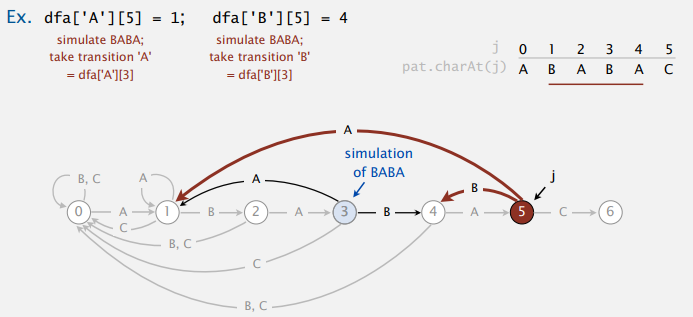这样，乍一看需要 j 步来模拟暴力算法中回退部分字符输入到 DFA，从而找到不匹配时的重启状态（像上图的状态 3）。但其实，我们可以花很小的成本来维护重启状态 X，不匹配时就能一下知道该转移到哪。其实也就是从 pat 开始匹配而已，具体可以看下面代码。

#### Construcing DFA For KMP: Java Implementation

``````public KMP(String pat) {
this.pat = pat;
M = pat.length();
dfa = new int[R][M];
dfa[pat.charAt(0)] = 1;            // 初始状态只要这一个转移，其它还是自己（0）
// 构造 dfa[][1..M - 1] 没有终结状态的转移
for (int X = 0, j = 1; j < M; j++) {
for (int c = 0; c < R; c++)
dfa[c][j] = dfa[c][X];        // copy mismatch cases
// 匹配时，状态和重启状态没关系，以当前状态为主，直接下一状态
dfa[pat.charAt(j)][j] = j + 1;    // set match case
// 从 pat 开始匹配
X = dfa[pat.charAt(j)][X];        // update restart state
}
}
``````

## Boyer-Moore

Boyer-Moore 算法采用启发式（heuritic）的方法处理不匹配的字符，从右向左扫描模式字符串（长度为 M）并将它和文本匹配，不匹配的时候最多可以跳过 M 个文本中的字符，在实际应用中近似能达到 \$N/M\$ 级别。例子：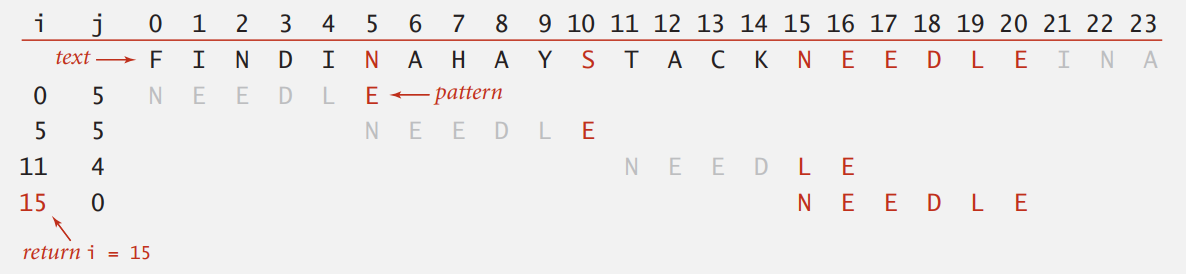1. 不匹配字符不在模式串中。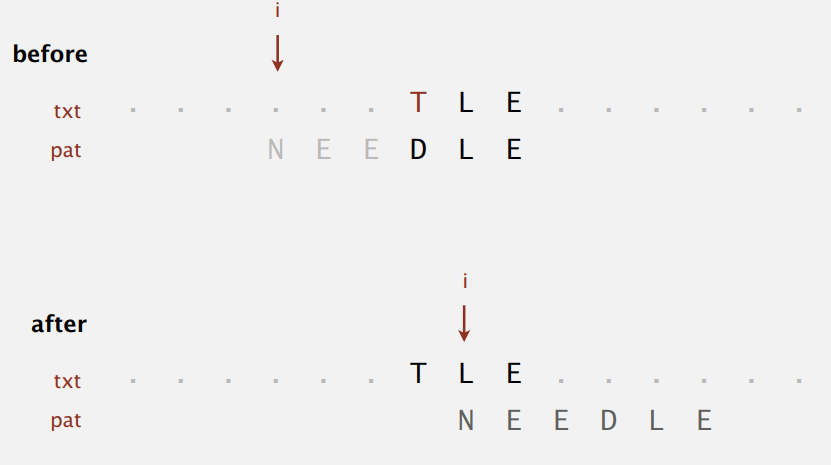这是最简单的情况，直接移动 M 位。

2. 不匹配字符在模式串中，情形一。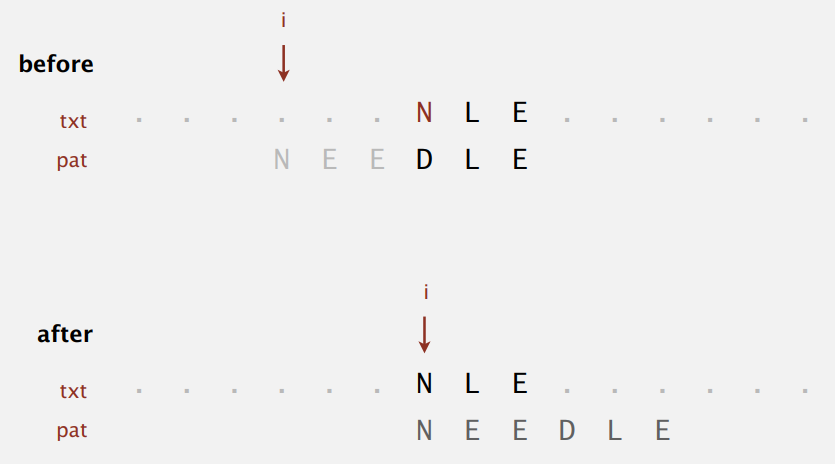将模式串中最右边的该字符和文本中的对齐。

3. 不匹配字符在模式串中，情形二。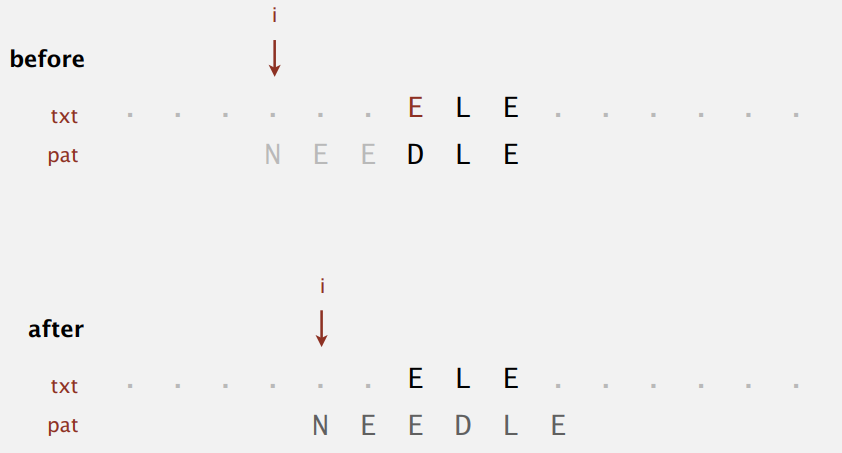这时要是按上面和最右边的对齐，模式串会往左边移动发生回退，移动位数是负数。所以这时没办法跳过很多字符，只能老实地右移一位。

``````rigth = new int[R];
for (int c = 0; c < R; c++)
right[c] = -1;    // 模式串中没有该字符则记为 -1
for (int j = 0; j < M; j++)
right[pat.charAt(j)] = j;
``````

### Boyer-Moore: Java Implementation

``````public int search(String txt) {
int N = txt.length();
int M = pat.length();
int skip;
for (int i = 0; i <= N - M; i += skip) {
skip = 0;
for (int j = M - 1; j >= 0; j--) {
if (pat.charAt(j) != txt.charAt(i + j)) {
// in case other term is nonpositive
skip = Math.max(1, j - right[txt.charAt(i + j)]);
}
}
if (skip == 0) return i;    // match
}
return N;
}
``````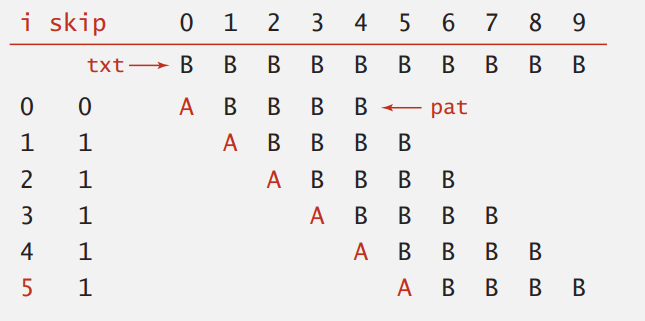## Rabin-Karp

Rabin-Karp 指纹字符串查找算法基于模数（modular）散列，直接上图：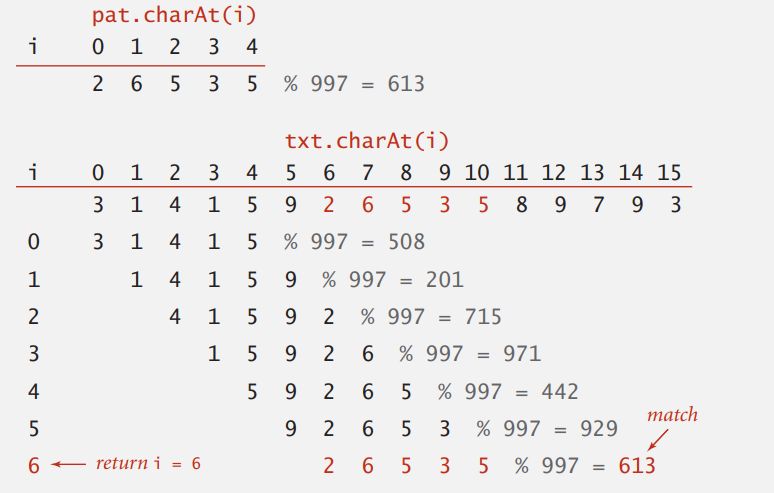\$x_{i} = c_{i}R^{M - 1} + c_{i + 1}R^{M - 2} + ... + c_{i + M - 1}R^{0}\$ (mod Q)

\$c_{i}\$ 表示第 i 位的字符，Q 就是一个很大的素数（也要注意不要溢出）。然后再用 Horner 方法（霍尔法则计算 n 次多项式）在线性时间内计算出来：

``````// Compute hash for M-digit key
private long hash(String key, int M) {
long h = 0;
for (int j = 0; j < M; j++)
h = (R * h + key.charAt(j)) % Q;
return h;
}
``````

\$x_{i} = c_{i}R^{M - 1} + c_{i + 1}R^{M - 2} + ... + c_{i + M - 1}R^{0}\$

\$x_{i + 1} = c_{i + 1}R^{M - 1} + c_{i + 2}R^{M - 2} + ... + c_{i + M}R^{0}\$

\$x_{i + 1} = (x_{i} - t_{i}R^{M - 1}) R + t_{i + M}\$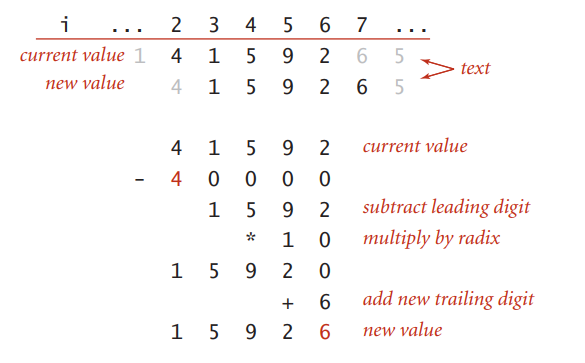### Rabin-Krap: Java Implementation

``````public class RabinKrap {
private long patHash;    // pattern hash value
private int M;           // pattern length
private long Q;          // modulus
private long RM;         // R^(M-1) % Q

public RabinKrap(String pat) {
R = 256;
M = pat.length();
patHash = hash(pat, M);
// a large prime
// but avoid overflow
Q = longRandomPrime();

RM = 1;
for (int i = 1; i <= M -1; i++)
RM = (R * RM) % Q;
}

public int search(String txt) {
int N = txt.length();
int txtHash = hash(txt, M);
if (patHash == txtHash) return 0;
for (int i = M; i < N; i++) {
txtHash = (txtHash + Q - RM * txt.charAt(i - M) % Q) % Q;
txtHash = (txtHash * R + txt.charAt(i)) % Q;
if (patHash == txtHash) return i - M + 1;    // Monte Carlo version
}
}
}
``````

Rabin-Karp 算法的优点是容易拓展，像是拓展到查找多模式，查找二维模式等，缺点是算术运算会比其它算法的字符比较慢等。

o

### osc_pn11u1x9#### 暂无文章

VB语言基础重要知识点12

2019/10/30
5
0

kuls
01/16
18
0

04/24
14
0

04/29
5
0
how to install mongodb in centos7

[root@xtwj88 ~]# cat /etc/yum.repos.d/mongodb-org-4.2.repo [mongodb-org-4.2]name=MongoDB Repositorybaseurl=https://repo.mongodb.org/yum/redhat/\$releasever/mongodb-org/4.2/x86......

qwfys
59分钟前
13
0